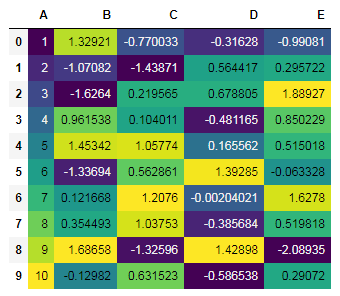﻿ Pandas styling: Write a Pandas program to display the dataframe in Heatmap style - w3resource# Pandas styling Exercises: Write a Pandas program to display the dataframe in Heatmap style

## Pandas styling: Exercise-11 with Solution

Create a dataframe of ten rows, four columns with random values. Write a Pandas program to display the dataframe in Heatmap style.

Sample Solution :

Python Code :

``````import pandas as pd
import numpy as np
import seaborn as sns

np.random.seed(24)
df = pd.DataFrame({'A': np.linspace(1, 10, 10)})
df = pd.concat([df, pd.DataFrame(np.random.randn(10, 4), columns=list('BCDE'))],
axis=1)
print("Original array:")
print(df)
print("\nDataframe - Heatmap style:")

cm = sns.light_palette("red", as_cmap=True)

``````

Original array:

```Original array:
A         B         C         D         E
0   1.0  1.329212 -0.770033 -0.316280 -0.990810
1   2.0 -1.070816 -1.438713  0.564417  0.295722
2   3.0 -1.626404  0.219565  0.678805  1.889273
3   4.0  0.961538  0.104011 -0.481165  0.850229
4   5.0  1.453425  1.057737  0.165562  0.515018
5   6.0 -1.336936  0.562861  1.392855 -0.063328
6   7.0  0.121668  1.207603 -0.002040  1.627796
7   8.0  0.354493  1.037528 -0.385684  0.519818
8   9.0  1.686583 -1.325963  1.428984 -2.089354
9  10.0 -0.129820  0.631523 -0.586538  0.290720

Dataframe - Heatmap style:
```

Sample Output:

``````

Python Code Editor:

Have another way to solve this solution? Contribute your code (and comments) through Disqus.

Next: Create a dataframe of ten rows, four columns with random values. Write a Pandas program to make a gradient color mapping on a specified column.

What is the difficulty level of this exercise?

﻿

## Python: Tips of the Day

Python: How do I concatenate two lists in Python?

You can use the + operator to combine them:

```listone = [1,2,3]
listtwo = [4,5,6]

joinedlist = listone + listtwo
```

Output:

```>>> joinedlist
[1,2,3,4,5,6]
```

Ref: https://bit.ly/2B8fL40